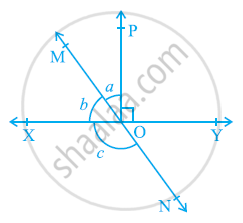Share

# In the given figure, lines XY and MN intersect at O. If ∠POY = 90° and a:b = 2 : 3, find c. - CBSE Class 9 - Mathematics

#### Question

In the given figure, lines XY and MN intersect at O. If ∠POY = 90° and a:b = 2 : 3, find c.#### Solution

Let the common ratio between a and b be x.

∴ a = 2x, and b = 3x

XY is a straight line, rays OM and OP stand on it.

∴ ∠XOM + ∠MOP + ∠POY = 180º

b + a + ∠POY = 180º

3x + 2x + 90º = 180º

5x = 90º

x = 18º

a = 2x = 2 × 18 = 36º

b = 3x= 3 ×18 = 54º

MN is a straight line. Ray OX stands on it.

∴ b + c = 180º (Linear Pair)

54º + c = 180º

c = 180º − 54º = 126º

∴ c = 126º

Is there an error in this question or solution?

#### APPEARS IN

NCERT Solution for Mathematics Class 9 (2018 to Current)
Chapter 6: Lines and Angles
Ex. 6.10 | Q: 2 | Page no. 97

#### Video TutorialsVIEW ALL 

Solution In the given figure, lines XY and MN intersect at O. If ∠POY = 90° and a:b = 2 : 3, find c. Concept: Pairs of Angles.
S